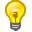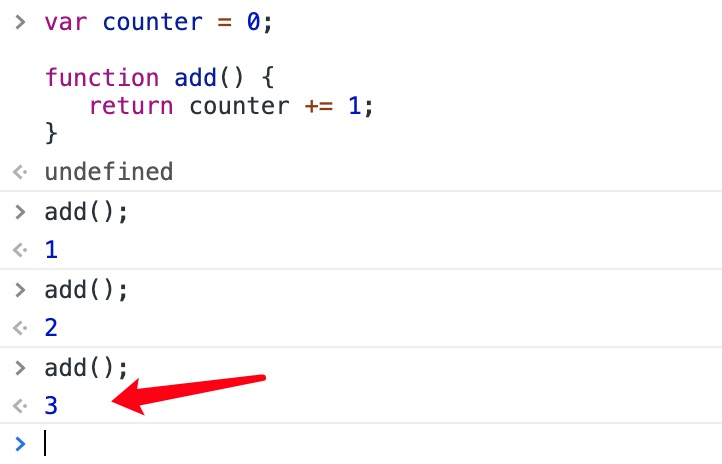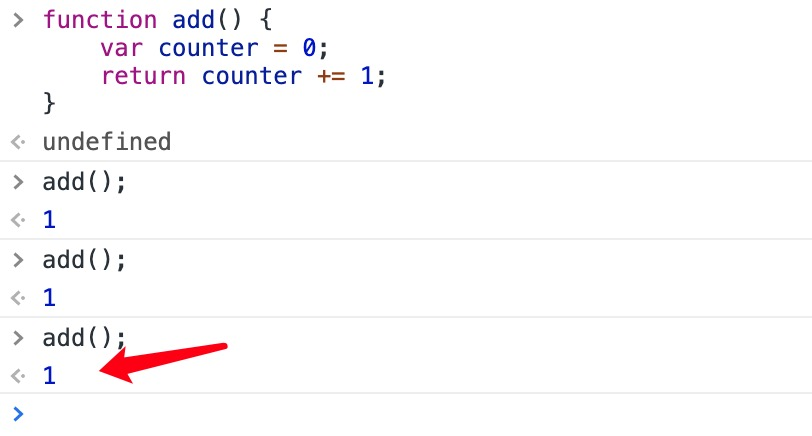# JS 一 函数定义

JavaScript 使用关键字 function 定义函数。

## 函数声明

function functionName(parameters) {
执行的代码
}分号是用来分隔可执行JavaScript语句。 由于函数声明不是一个可执行语句，所以不以分号结束。

## 函数表达式

JavaScript 函数可以通过一个表达式定义。

## 实例

var x = function (a, b) {return a * b};

## 实例

var x = function (a, b) {return a * b};
var z = x(4, 3);上述函数以分号结尾，因为它是一个执行语句。

## 实例

var myFunction = new Function("a", "b", "return a * b");

var x = myFunction(4, 3);

## 实例

var myFunction = function (a, b) {return a * b};

var x = myFunction(4, 3);在 JavaScript 中，很多时候，你需要避免使用 new 关键字。

## 函数提升（Hoisting）

myFunction(5);

function myFunction(y) {
return y * y;
}

## 实例

(function () {
var x = "Hello!!";      // 我将调用自己
})();

## 函数可作为一个值使用

JavaScript 函数作为一个值使用：

## 实例

function myFunction(a, b) {
return a * b;
}

var x = myFunction(4, 3);

JavaScript 函数可作为表达式使用：

## 实例

function myFunction(a, b) {
return a * b;
}

var x = myFunction(4, 3) * 2;

## 函数是对象

JavaScript 函数有 属性 和 方法

arguments.length 属性返回函数调用过程接收到的参数个数：

## 实例

function myFunction(a, b) {
return arguments.length;
}

toString() 方法将函数作为一个字符串返回:

## 实例

function myFunction(a, b) {
return a * b;
}

var txt = myFunction.toString();函数定义作为对象的属性，称之为对象方法。 函数如果用于创建新的对象，称之为对象的构造函数。

## 箭头函数

ES6 新增了箭头函数。

(参数1, 参数2, …, 参数N) => { 函数声明 }

(参数1, 参数2, …, 参数N) => 表达式(单一)
// 相当于：(参数1, 参数2, …, 参数N) =>{ return 表达式; }

(单一参数) => {函数声明}

() => {函数声明}

## 实例

// ES5
var x = function(x, y) {
return x * y;
}

// ES6
const x = (x, y) => x * y;

## 实例

const x = (x, y) => { return x * y };

# JS 二 函数参数

JavaScript 函数对参数的值没有进行任何的检查。

## 函数显式参数(Parameters)与隐式参数(Arguments)

functionName(parameter1, parameter2, parameter3) {
// 要执行的代码……
}

## 参数规则

JavaScript 函数定义显式参数时没有指定数据类型。

JavaScript 函数对隐式参数没有进行类型检测。

JavaScript 函数对隐式参数的个数没有进行检测。

## 默认参数

ES5 中如果函数在调用时未提供隐式参数，参数会默认设置为： undefined

## 实例(ES5)

function myFunction(x, y) {
if (y === undefined) {
y = 0;
}
}

## 实例(ES5)

function myFunction(x, y) {
y = y || 0;
}如果y已经定义 ， y || 返回 y, 因为 y 是 true, 否则返回 0, 因为 undefined 为 false。

### ES6 函数可以自带参数

ES6 支持函数带有默认参数，就判断 undefined 和 || 的操作：

## 实例（ES6）

function myFunction(x, y = 10) {
// y is 10 if not passed or undefined
return x + y;
}

myFunction(0, 2) // 输出 2
myFunction(5); // 输出 15, y 参数的默认值

## arguments 对象

JavaScript 函数有个内置的对象 arguments 对象。

argument 对象包含了函数调用的参数数组。

## 实例

x = findMax(1, 123, 500, 115, 44, 88);

function findMax() {
var i, max = arguments;

if(arguments.length < 2) return max;

for (i = 0; i < arguments.length; i++) {
if (arguments[i] > max) {
max = arguments[i];
}
}
return max;
}

## 实例

x = sumAll(1, 123, 500, 115, 44, 88);

function sumAll() {
var i, sum = 0;
for (i = 0; i < arguments.length; i++) {
sum += arguments[i];
}
return sum;
}

## 通过值传递参数

JavaScript 隐式参数通过值来传递：函数仅仅只是获取值。

# JS 三 函数调用

JavaScript 函数有 4 种调用方式。

## this 关键字注意 this 是保留关键字，你不能修改 this 的值。

## 实例

function myFunction(a, b) {
return a * b;
}
myFunction(10, 2);           // myFunction(10, 2) 返回 20

myFunction() 和 window.myFunction() 是一样的：

## 实例

function myFunction(a, b) {
return a * b;
}
window.myFunction(10, 2);    // window.myFunction(10, 2) 返回 20这是调用 JavaScript 函数常用的方法， 但不是良好的编程习惯 全局变量，方法或函数容易造成命名冲突的bug。

## 实例

function myFunction() {
return this;
}
myFunction();                // 返回 window 对象函数作为全局对象调用，会使 this 的值成为全局对象。 使用 window 对象作为一个变量容易造成程序崩溃。

## 实例

var myObject = {
firstName:"John",
lastName: "Doe",
fullName: function () {
return this.firstName + " " + this.lastName;
}
}
myObject.fullName();         // 返回 "John Doe"

fullName 方法是一个函数。函数属于对象。 myObject 是函数的所有者。

this对象，拥有 JavaScript 代码。实例中 this 的值为 myObject 对象。

## 实例

var myObject = {
firstName:"John",
lastName: "Doe",
fullName: function () {
return this;
}
}
myObject.fullName();          // 返回 [object Object] (所有者对象)函数作为对象方法调用，会使得 this 的值成为对象本身。

## 实例

// 构造函数:
function myFunction(arg1, arg2) {
this.firstName = arg1;
this.lastName  = arg2;
}

// This    creates a new object
var x = new myFunction("John","Doe");
x.firstName;                             // 返回 "John"构造函数中 this 关键字没有任何的值。this 的值在函数调用实例化对象(new object)时创建。

## 作为函数方法调用函数

call() 和 apply() 是预定义的函数方法。 两个方法可用于调用函数，两个方法的第一个参数必须是对象本身。

## 实例

function myFunction(a, b) {
return a * b;
}
myObject = myFunction.call(myObject, 10, 2);     // 返回 20

## 实例

function myFunction(a, b) {
return a * b;
}
myArray = [10, 2];
myObject = myFunction.apply(myObject, myArray);  // 返回 20通过 call() 或 apply() 方法你可以设置 this 的值, 且作为已存在对象的新方法调用。

this 是 JavaScript 语言的一个关键字。

function test() {
this.x = 1;
}

<script>
var myObject, myArray;
myObject={
name: "hahaha ",
hsk: "en"
};
function myFunction(a, b) {
return this.name +this.hsk;
}
myArray = [10, 2]
myObject = myFunction.apply(myObject, myArray);
document.getElementById("demo").innerHTML = myObject;
</script>

var value = '俊俊吃饭饭'

var obj = {
fun: function () {
console.log(this)
}
}

obj.fun.call(value) //这里的this不是window，也不是obj,这里的this就是value的值:"俊俊吃饭饭"

var value = '俊俊吃饭饭'

var obj = {
fun: function () {
console.log(this)
}
}

obj.fun.call(null)  //window
obj.fun.call(undefined)  //window

# JS 四 闭包

JavaScript 变量可以是局部变量或全局变量。

## 实例

function myFunction() {
var a = 4;
return a * a;
}

## 实例

var a = 4;
function myFunction() {
return a * a;
}变量声明时如果不使用 var 关键字，那么它就是一个全局变量，即便它在函数内定义。

## 实例

var counter = 0;

return counter += 1;
}

// 计数器现在为 3## 实例

function add() {
var counter = 0;
return counter += 1;
}

// 本意是想输出 3, 但事与愿违，输出的都是 1 !JavaScript 内嵌函数可以解决该问题。

## JavaScript 内嵌函数

JavaScript 支持嵌套函数。嵌套函数可以访问上一层的函数变量。

## 实例

function add() {
var counter = 0;
function plus() {counter += 1;}
plus();
return counter;
}

## 实例

var add = (function () {
var counter = 0;
return function () {return counter += 1;}
})();

// 计数器为 3

## 实例解析闭包是一种保护私有变量的机制，在函数执行时形成私有的作用域，保护里面的私有变量不受外界干扰。 直观的说就是形成一个不销毁的栈环境。
10-31
07-101万+

02-212810
06-03268
01-291326
04-14276
04-079109
03-132029
11-215194
01-032416
12-134676
01-272167
01-271310
06-159622
03-191万+
08-06421
08-062万+
03-28114

### “相关推荐”对你有帮助么？

•非常没帮助
•没帮助
•一般
•有帮助
•非常有帮助Mirst

¥2 ¥4 ¥6 ¥10 ¥20余额支付 (余额：-- )扫码支付获取中扫码支付点击重新获取扫码支付1.余额是钱包充值的虚拟货币，按照1:1的比例进行支付金额的抵扣。
2.余额无法直接购买下载，可以购买VIP、C币套餐、付费专栏及课程。余额充值